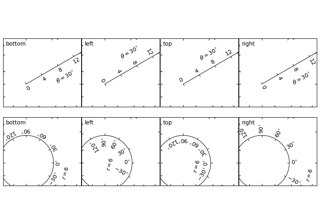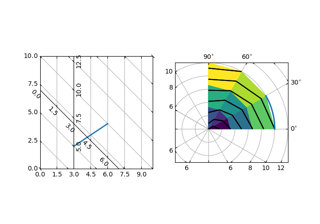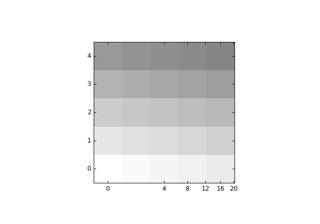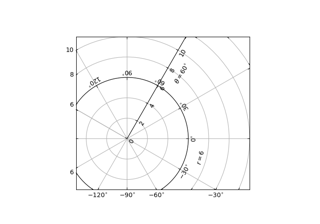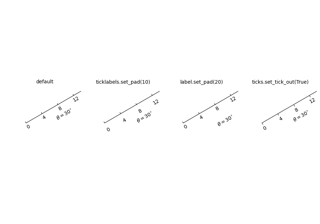# mpl_toolkits.axisartist.grid_finder.ExtremeFinderSimple¶

class mpl_toolkits.axisartist.grid_finder.ExtremeFinderSimple(nx, ny)[source]

Bases: object

A helper class to figure out the range of grid lines that need to be drawn.

Parameters: nx, nyintThe number of samples in each direction.
__call__(transform_xy, x1, y1, x2, y2)[source]

Compute an approximation of the bounding box obtained by applying transform_xy to the box delimited by (x1, y1, x2, y2).

The intended use is to have (x1, y1, x2, y2) in axes coordinates, and have transform_xy be the transform from axes coordinates to data coordinates; this method then returns the range of data coordinates that span the actual axes.

The computation is done by sampling nx * ny equispaced points in the (x1, y1, x2, y2) box and finding the resulting points with extremal coordinates; then adding some padding to take into account the finite sampling.

As each sampling step covers a relative range of 1/nx or 1/ny, the padding is computed by expanding the span covered by the extremal coordinates by these fractions.

__dict__ = mappingproxy({'__module__': 'mpl_toolkits.axisartist.grid_finder', '__doc__': '\n A helper class to figure out the range of grid lines that need to be drawn.\n ', '__init__': <function ExtremeFinderSimple.__init__>, '__call__': <function ExtremeFinderSimple.__call__>, '_add_pad': <function ExtremeFinderSimple._add_pad>, '__dict__': <attribute '__dict__' of 'ExtremeFinderSimple' objects>, '__weakref__': <attribute '__weakref__' of 'ExtremeFinderSimple' objects>, '__annotations__': {}})
__init__(nx, ny)[source]
Parameters: nx, nyintThe number of samples in each direction.
__module__ = 'mpl_toolkits.axisartist.grid_finder'
__weakref__

list of weak references to the object (if defined)

## Examples using mpl_toolkits.axisartist.grid_finder.ExtremeFinderSimple¶